Courses

# Propagation Delay Calculation of CMOS Inverter - Electronics & Communication Engineering Electrical Engineering (EE) Notes | EduRev

## VLSI System Design

Created by: Machine Experts

## Electrical Engineering (EE) : Propagation Delay Calculation of CMOS Inverter - Electronics & Communication Engineering Electrical Engineering (EE) Notes | EduRev

The document Propagation Delay Calculation of CMOS Inverter - Electronics & Communication Engineering Electrical Engineering (EE) Notes | EduRev is a part of the Electrical Engineering (EE) Course VLSI System Design.
All you need of Electrical Engineering (EE) at this link: Electrical Engineering (EE)

Objectives

In this lecture you will learn the following

• Few Definitions
• Quick Estimates
• Rise and Fall times Calculation

16.1 Few Definitions

Before calculating the propagation delay of CMOS Inverter, we will define some basic terms-

• Switching speed - limited by time taken to charge and discharge, CL .
• Rise time, tr : waveform to rise from 10% to 90% of its steady state value
• Fall time tf, : 90% to 10% of steady state value
• Delay time, td : time difference between input transition (50%) and 50% output level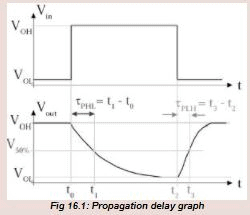The propagation delay tp of a gate defines how quickly it responds to a change at its inputs, it expresses the delay experienced by a signal when passing through a gate. It is measured between the 50% transition points of the input and output waveforms as shown in the figure 16.1 for an inverting gate. The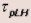defines the response time of the gate for a low to high output transition, while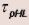refers to a high to low transition. The propagation delay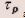as the average of the two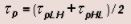16.2 Quick Estimates:

We will give an example of how to calculate quick estimate. From ffig 16.22, we can write following equations..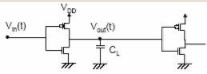Fig 16.21: Example CMOS Inverter Circuit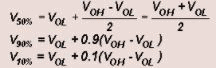From figure 16.21, when Vin = 0 the capacitor CL charges through the P-MOS, and when Vin = 5 the capacitor discharges through the N-MOS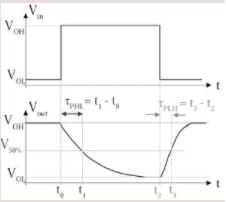Fig 16.22 : Propagation Delay of above

The capacitor current is -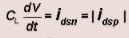MOS Circuit

From this the delay times can be derived as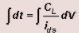The expressions for the propagation delays as denoted in the figure (16.22) can be easily seen to be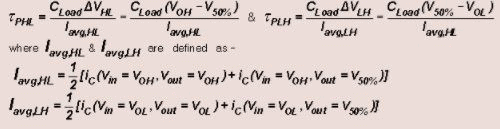16.3 Rise and Fall Times

Figure 16.21 shows the familiar CMOS inverter with a capacity load CL that represents the load capacitance (input of next gates, output of this gate and routing). Of interest is the voltage waveform Vout(t) when the input is driven by a step waveform, Vin(t)as shown in figure 16.22 .

Figure 16.31 shows the trajectory of the n-transistor operating point as the input voltage, Vin(t), changes from 0V to VDD. Initially, the end-device is cutt-off and the load capacitor is charged to VDD. This illustrated by X1 on the characteristic curve. Application of a step voltage (VGS = VDD) at the input of the inverter changes the operating point to X2. From there onwards the trajectory moves on the VGS = VDD characteristic curve towards point X3 at the origin.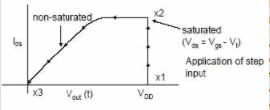Fig 16.31: trjectory of n-transistor operating point

Thus it is evident that the fall time consists of two intervals;

1.tf1=period during which the capacitor voltage, Vout, drops from 0.9VDD to (VDD– Vtn)
2. tf2=period during which the capacitor voltage, Vout, drops from (VDD – Vtn ) to 0.1VDD.
The equivalent circuits that illustrate the above behavior are show in figure (16.32 & 16.33 ).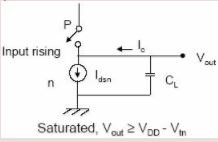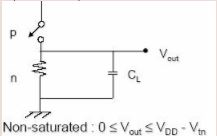Figure 16.32: Equivalent circuit for showing behav. of tf1                   Figure 16.33: Equivalent circuit for showing behav. of tf2

As we saw in last section, the delay periods can be derived using the general equationFrom figure (16.32) while in saturation,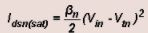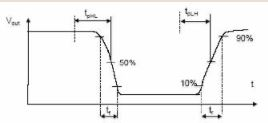Fig 16.34: Rise and Fall time graph

Integrating from t = t1, corresponding to Vout=0.9 VDD, to t = tcorresponding to Vout=(VDD-Vtn) results in,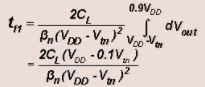When the n-device begins to operate in the linear region, the

discharge current is no longer constant. The time tf1 taken to discharge the capacitor voltage from (VDD-Vtn) to 0.1VDD can be obtained as before. In linear region,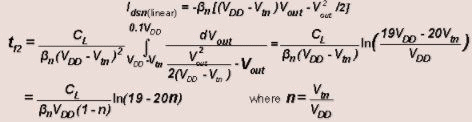Thus the complete term for the fall time is,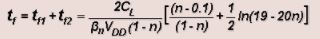The fall time tf can be approximated as,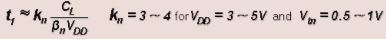From this expression we can see that the delay is directly proportional to the load capacitance. Thus to achieve high speed circuits one has to minimize the load capacitance seen by a gate. Secondly it is inversely proportion to the supply voltage i.e. as the supply voltage is raised the delay time is reduced. Finally, the delay is proportional to the βn of the driving transistor so increasing the width of a transistor decreases the delay.

Due to the symmetry of the CMOS circuit the rise time can be similarly obtained as;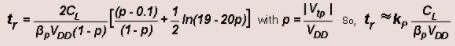For equally sized n and p transistors (where βn=2βp) tf=tr

Thus the fall time is faster than the rise time primarily due to different carrier mobilites associated with the p and n devices thus if we want tf=tr we need to make βn/βp =1. This implies that the channel width for the p-device must be increased to approximately 2 to 3 times that of the n-device.

The propagation delays if calculated as indicated before turn out to be,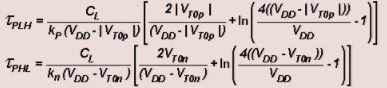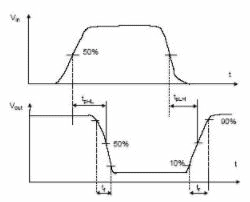Figure 16.35: Rise and Fall time graph of Output w.r.t Input

If we consider the rise time and fall time of the input signal as well, as shown in the fig 16.35 we have,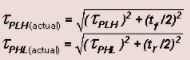These are the rms values for the propagation delays.

Offer running on EduRev: Apply code STAYHOME200 to get INR 200 off on our premium plan EduRev Infinity!

35 docs|28 tests

,

,

,

,

,

,

,

,

,

,

,

,

,

,

,

,

,

,

,

,

,

;